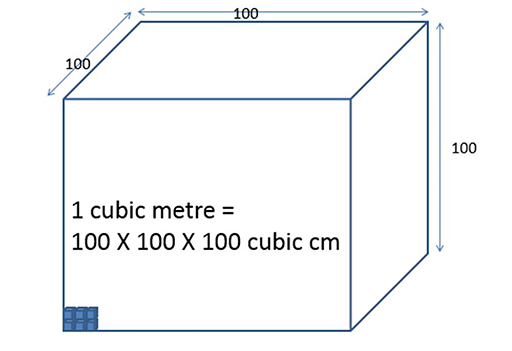Education & Development

### Become an OU studentTeaching mathematics

Start this free course now. Just create an account and sign in. Enrol and complete the course for a free statement of participation or digital badge if available.

# 4.4 Converting cubic centimetres into cubic metres

If you imagine a box which is one cubic metre then 100 cubic centimetres would line up along its edge.

You could fit 100 rows of these 100 cubic centimetres in one layer.

There would be 100 layers of cubic centimetres in the cubic metre.

That’s 100 × 100 × 100 = 1 000 000 cubic centimetres in one cubic metre (Figure 15).Figure 15 A cubic metre